# Test: Engineering Mathematics- 6

## 10 Questions MCQ Test RRB JE for Computer Science Engineering | Test: Engineering Mathematics- 6

Description
Attempt Test: Engineering Mathematics- 6 | 10 questions in 30 minutes | Mock test for Computer Science Engineering (CSE) preparation | Free important questions MCQ to study RRB JE for Computer Science Engineering for Computer Science Engineering (CSE) Exam | Download free PDF with solutions
QUESTION: 1

### What is the value of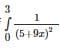Solution: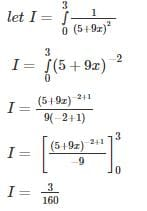QUESTION: 2

###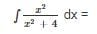Solution: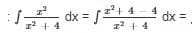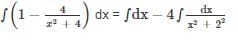= x – 2 tan –1 (x/2)+ C

QUESTION: 3

### The value of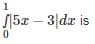Solution: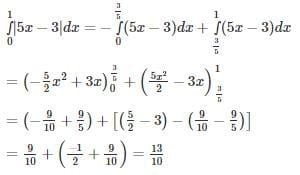QUESTION: 4

∫e{f(x) + f′(x)} dx is equal to

Solution: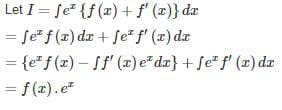QUESTION: 5

Find the value of the given function f?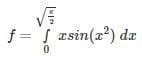Solution: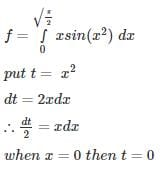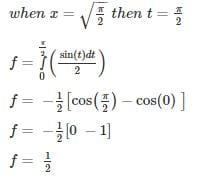QUESTION: 6

What it the value of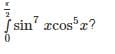Solution: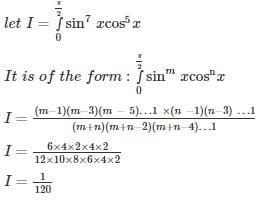QUESTION: 7

Find the value of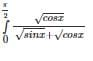Solution: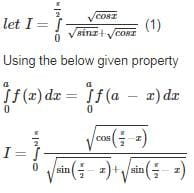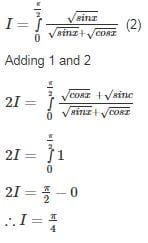QUESTION: 8

If x = -1 and x = 2 are extreme points of f(x) = a log |x| + βx2 + x then

Solution:

(f'(x)) = 0

a/x + 2bx +1= 0

at  x=-1,  -a - 2b + 1 = 0

a + 2b = 1 ..........(1)

At x=2,  a/2 + 4b + 1 = 0

a + 8b = -2 ..........(2)

subtracting (1) & (2),   b=-1/2

=> a=2

QUESTION: 9

Solve the integral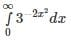Solution: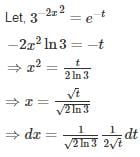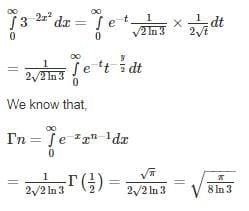*Answer can only contain numeric values
QUESTION: 10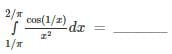Solution: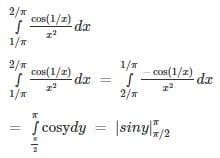= 0 - 1 = - 1Use Code STAYHOME200 and get INR 200 additional OFF Use Coupon Code